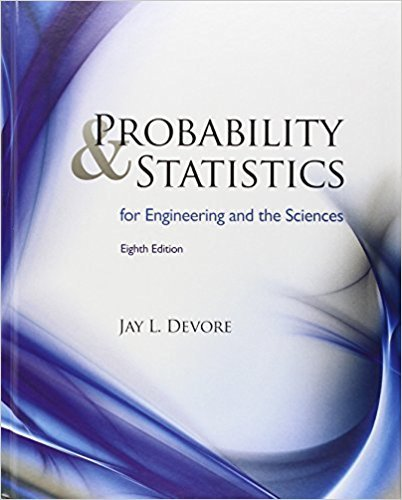×
Get Full Access to Probability And Statistics For Engineering And The Sciences - 8 Edition - Chapter 9 - Problem 30
Get Full Access to Probability And Statistics For Engineering And The Sciences - 8 Edition - Chapter 9 - Problem 30

×

# The article “Flexure of Concrete Beams Reinforced withISBN: 9780538733526 61

## Solution for problem 30 Chapter 9

Probability and Statistics for Engineering and the Sciences | 8th Edition

• Textbook Solutions
• 2901 Step-by-step solutions solved by professors and subject experts
• Get 24/7 help from StudySoup virtual teaching assistantsProbability and Statistics for Engineering and the Sciences | 8th Edition

4 5 1 244 Reviews
18
2
Problem 30

The article “Flexure of Concrete Beams Reinforced with Advanced Composite Orthogrids” (J. of Aerospace Engr., 1997: 7–15) gave the accompanying data on ultimate load (kN) for two different types of beams. a. Assuming that the underlying distributions are normal, calculate and interpret a 99% CI for the difference between true average load for the fiberglass beams and that for the carbon beams. b. Does the upper limit of the interval you calculated in part (a) give a 99% upper confidence bound for the difference between the two m’s? If not, calculate such a bound. Does it strongly suggest that true average load for the carbon beams is more than that for the fiberglass beams? Explain.

Step-by-Step Solution:
Step 1 of 3

I. 01.23.2017 bio lecture chapter two A. the chemical basis of life ELEMENT: fundamental substance; can’t break down to other substances by chemical reaction (rxn) COMPOUND: 2+ elements (atoms) chemically bounded e.g. NaCl (sodium chloride) MOLECULE: 2+ atoms, same or different Life on Earth requires about 24 elements; oxygen greatest by weight. Trace elements (less than 0.01% ((1/100))): boron, chromium cobalt, iron, zinc, etc. -can contribute to structure, allow enzymes to work, etc. iron- critical to how oxygen is transported in blood. Fluorine- bones each element corresponds to an ATOM mass of atom: almost all due to PROTONS and NEUTRONS (e- weight almost nothing) elements are described by number of subatomic particles for most atoms

Step 2 of 3

Step 3 of 3

##### ISBN: 9780538733526

Unlock Textbook Solution